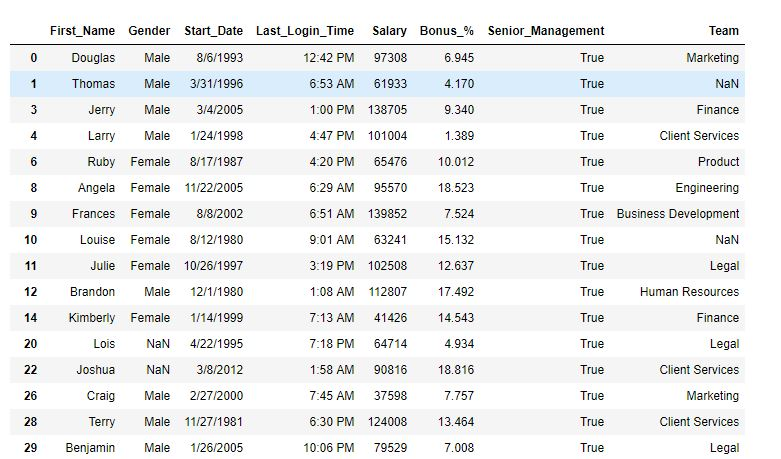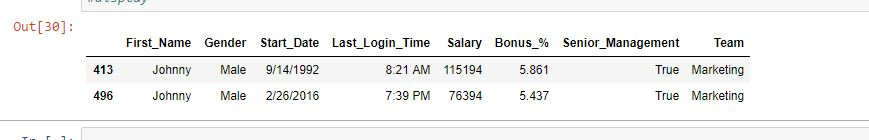# Python | Filtering data with Pandas .query() method

• Difficulty Level : Basic
• Last Updated : 23 Aug, 2019

Python is a great language for doing data analysis, primarily because of the fantastic ecosystem of data-centric Python packages. Pandas is one of those packages that makes importing and analyzing data much easier.

Analyzing data requires a lot of filtering operations. Pandas provide many methods to filter a Data frame and `Dataframe.query()` is one of them.

Syntax: DataFrame.query(expr, inplace=False, **kwargs)

Parameters:
expr: Expression in string form to filter data.
inplace: Make changes in the original data frame if True
kwargs: Other keyword arguments.

Return type: Filtered Data frame

Note: `Dataframe.query()` method only works if the column name doesn’t have any empty spaces. So before applying the method, spaces in column names are replaced with ‘_’

Example #1: Single condition filtering

In this example, the data is filtered on the basis of single condition. Before applying the query() method, the spaces in column names have been replaced with ‘_’.

 `# importing pandas package``import` `pandas as pd`` ` `# making data frame from csv file ``data ``=` `pd.read_csv(``"employees.csv"``)`` ` `# replacing blank spaces with '_' ``data.columns ``=``[column.replace(``" "``, ``"_"``) ``for` `column ``in` `data.columns]`` ` `# filtering with query method``data.query(``'Senior_Management == True'``, inplace ``=` `True``)`` ` `# display``data`

Output:
As shown in the output image, the data now only have rows where Senior Management is True.Example #2: Multiple condition filtering

In this example, dataframe has been filtered on multiple conditions. Before applying the query() method, the spaces in column names have been replaced with ‘_’.

 `# importing pandas package``import` `pandas as pd`` ` `# making data frame from csv file ``data ``=` `pd.read_csv(``"employees.csv"``)`` ` `# replacing blank spaces with '_' ``data.columns ``=``[column.replace(``" "``, ``"_"``) ``for` `column ``in` `data.columns]`` ` `# filtering with query method``data.query('Senior_Management ``=``=` `True` `            ``and` `Gender ``=``=``"Male"` `and` `Team ``=``=``"Marketing"` `            ``and` `First_Name ``=``=``"Johnny"``', inplace ``=` `True``)`` ` `# display``data`

Output:
As shown in the output image, only two rows have been returned on the basis of filters applied.My Personal Notes arrow_drop_up